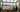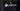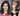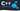Hurry Up !! Check Out Todays Deal

# OpenCV - Image Segmentation using Watershed algorithm C++

The watershed technique is a well-known segmentation algorithm that is particularly good for extracting touching or overlapping items in photographs.

## Marker-based Watershed algorithm

We must start with user-defined markers when using the watershed method. These markers can be manually defined using point-and-click, or they can be defined automatically or heuristically using thresholding and/or morphological processes.

The watershed technique treats pixels in our input image as local elevation (called topography) based on these markers — the method "floods" valleys from the markers outwards until the valleys of various markers meet. The markers must be set accurately in order to produce an accurate watershed segmentation.

We used an OpenCV marker-based watershed technique to designate which valley points should be merged and which should not. It is an interactive image segmentation rather than an automatic image segmentation.

## Theory

Any grayscale image can be regarded as a topographic surface, with peaks and hills denoting high intensity and valleys denoting low intensity. You begin by filling isolated valleys (local minima) with a variety of coloured water (labels). River from separate valleys, plainly of different colours, will start to blend as the water rises, depending on the neighbouring peaks (gradients). To avoid this, you construct barriers where water meets water. You keep filling the water and erecting obstacles until all of the peaks are submerged. The segmentation outcome is then determined by the obstacles you created. The watershed's "philosophy" is as follows. You may learn more about watershed on the CMM website, which includes animations.

However, due to noise or other anomalies in the image, this method produces an oversegmented result. As a result, OpenCV created a marker-based watershed method that allows you to select which valley points should be merged and which should not. It's a segmentation of an image that you may interact with. What we do is assign distinct labels to the objects we are familiar with. Label the zone that we are certain is the foreground or object with one colour (or intensity), the region that we are certain is the background or non-object with another colour, and the region that we are unsure about with 0.That's where we'll put our marker. After that, use the watershed algorithm. The bounds of objects will have a value of -1, and our marker will be updated with the labels we provided.

## Implementing Watershed Algorithm Using OpenCV and C++

``` if(argc < 2) { std::cerr << "Error\n"; std::cerr << "Provide Input Image:\n<program> <inputimage>\n"; return -1; } cv::Mat original_img = cv::imread(argv); if(original_img.empty()) { std::cerr << "Error\n"; std::cerr << "Cannot Read Image\n"; return -1; } ```

### 2. Applying Filter to remove noise from the image

``` cv::Mat shifted; cv::pyrMeanShiftFiltering(original_img,shifted,21,51); showImg("Mean Shifted",shifted); ```

Mean shift blur is a type of image edge preservation filtering algorithm that is frequently used to eliminate noise before image watershed segmentation, which can considerably improve the watershed segmentation effect.

### 3. Converting the original image to grayscale and binary image

``` cv::Mat gray_img; cv::cvtColor(original_img,gray_img,cv::COLOR_BGR2GRAY); showImg("GrayIMg",gray_img); cv::Mat bin_img; cv::threshold(gray_img,bin_img,0,255,cv::THRESH_BINARY | cv::THRESH_OTSU); showImg("thres img",bin_img); ```

### 4. Finding Sure Background of Image

In this step we find the area in the image which is sure to us that it is image background.

```void getBackground(const cv::Mat& source,cv::Mat& dst) { cv::dilate(source,dst,cv::Mat::ones(3,3,CV_8U)); //Kernel 3x3 } ```

### 5. Finding Sure Foreground of Image

For finding foreground of an image we use distance transform algorithm

```void getForeground(const cv::Mat& source,cv::Mat& dst) { cv::distanceTransform(source,dst,cv::DIST_L2,3,CV_32F); cv::normalize(dst, dst, 0, 1, cv::NORM_MINMAX); }```

### 6. Finding Marker

Before applying watershed algorithm we need markers. For this, we will use `findContour()` function provided in opencv to find marker in the image.

``` void findMarker(const cv::Mat& sureBg,cv::Mat& markers, std::vector<std::vector<cv::Point>>& contours) { cv::findContours(sureBg,contours,cv::RETR_EXTERNAL, cv::CHAIN_APPROX_SIMPLE); // Draw the foreground markers for (size_t i = 0,size = contours.size(); i < size; i++) drawContours(markers, contours, static_cast<int>(i), cv::Scalar(static_cast<int>(i)+1), -1); } ```

### 7. Applying Watershed algorithm

``` cv::watershed(original_img,markers); cv::Mat mark; markers.convertTo(mark, CV_8U); cv::bitwise_not(mark, mark); //Convert white to black and black to white showImg("MARKER",mark);```

## Code

``` #include <iostream> #include "opencv2/imgcodecs.hpp" #include "opencv2/highgui.hpp" #include "opencv2/imgproc.hpp" #include <vector> void showImg(const std::string& windowName,const cv::Mat& img){ cv::imshow(windowName,img); } void getBackground(const cv::Mat& source,cv::Mat& dst) { cv::dilate(source,dst,cv::Mat::ones(3,3,CV_8U)); //Kernel 3x3 } void getForeground(const cv::Mat& source,cv::Mat& dst) { cv::distanceTransform(source,dst,cv::DIST_L2,3,CV_32F); cv::normalize(dst, dst, 0, 1, cv::NORM_MINMAX); } void findMarker(const cv::Mat& sureBg,cv::Mat& markers, std::vector<std::vector<cv::Point>>& contours) { cv::findContours(sureBg,contours,cv::RETR_EXTERNAL, cv::CHAIN_APPROX_SIMPLE); // Draw the foreground markers for (size_t i = 0,size = contours.size(); i < size; i++) drawContours(markers, contours, static_cast<int>(i), cv::Scalar(static_cast<int>(i)+1), -1); } void getRandomColor(std::vector<cv::Vec3b>& colors,size_t size) { for (int i = 0; i < size ; ++i) { int b = cv::theRNG().uniform(0, 256); int g = cv::theRNG().uniform(0, 256); int r = cv::theRNG().uniform(0, 256); colors.emplace_back(cv::Vec3b((uchar)b, (uchar)g, (uchar)r)); } } int main (int argc,char** argv) { if(argc < 2) { std::cerr << "Error\n"; std::cerr << "Provide Input Image:\n<program> <inputimage>\n"; return -1; } cv::Mat original_img = cv::imread(argv); if(original_img.empty()) { std::cerr << "Error\n"; std::cerr << "Cannot Read Image\n"; return -1; } cv::Mat shifted; cv::pyrMeanShiftFiltering(original_img,shifted,21,51); showImg("Mean Shifted",shifted); cv::Mat gray_img; cv::cvtColor(original_img,gray_img,cv::COLOR_BGR2GRAY); showImg("GrayIMg",gray_img); cv::Mat bin_img; cv::threshold(gray_img,bin_img,0,255,cv::THRESH_BINARY | cv::THRESH_OTSU); showImg("thres img",bin_img); cv::Mat sure_bg; getBackground(bin_img,sure_bg); showImg("Sure Background",sure_bg); cv::Mat sure_fg; getForeground(bin_img,sure_fg); showImg("Sure ForeGround",sure_fg); cv::Mat markers = cv::Mat::zeros(sure_bg.size(),CV_32S); std::vector<std::vector<cv::Point>> contours; findMarker(sure_bg,markers,contours); cv::circle(markers, cv::Point(5, 5), 3, cv::Scalar(255), -1); //Drawing Circle around the marker cv::watershed(original_img,markers); cv::Mat mark; markers.convertTo(mark, CV_8U); cv::bitwise_not(mark, mark); //Convert white to black and black to white showImg("MARKER",mark); /* Highliting Markers in the image */ std::vector<cv::Vec3b> colors; getRandomColor(colors,contours.size()); // Create the result image cv::Mat dst = cv::Mat::zeros(markers.size(), CV_8UC3); // Fill labeled objects with random colors for (int i = 0; i < markers.rows; i++) { for (int j = 0; j < markers.cols; j++) { int index = markers.at<int>(i,j); if (index > 0 && index <= static_cast<int>(contours.size())) dst.at<cv::Vec3b>(i,j) = colors[index-1]; } } showImg("Final Result",dst); cv::waitKey(0); return 0; }```

### Realate Post#### PyTorch tutorial - Creating Convolutional Neural Network 

In this tutorial, we'll show you how to design convolutional neural network (CNN) models Create CNN models to solve a classification problem.#### OpenCV - Understanding Canny Edge Detection Using C++

Learn how to use OpenCV to detect edges.In OpenCV, experiment with various edge detection algorithms such as Canny.#### OpenCV - Face Recognition using LBPH Classifier in C++

In this tutorial we will walk through creating and trainning our own model which will recognize different faces using LBPHFaceRecognizer#### Parallel Programming with C++

A performance optimization lesson will teach you about C++17 parallel algorithms and how to improve C++ code performance using this library and the Intel Parallel STL